Download Presentation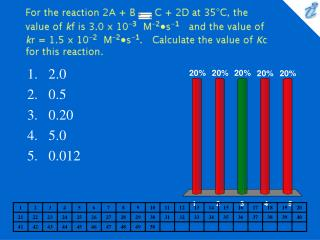2.0 0.5 0.20 5.0 0.012

# 2.0 0.5 0.20 5.0 0.012 - PowerPoint PPT Presentation

For the reaction 2A + B {image} C + 2D at 35&#176;C, the value of kf is 3.0 x 10-3 M-2&#8226;s-1 and the value of kr = 1.5 x 10-2 M-2&#8226;s-1. Calculate the value of Kc for this reaction. 2.0 0.5 0.20 5.0 0.012.I am the owner, or an agent authorized to act on behalf of the owner, of the copyrighted work described.
Download Presentation## 2.0 0.5 0.20 5.0 0.012

An Image/Link below is provided (as is) to download presentation

Download Policy: Content on the Website is provided to you AS IS for your information and personal use and may not be sold / licensed / shared on other websites without getting consent from its author.While downloading, if for some reason you are not able to download a presentation, the publisher may have deleted the file from their server.

- - - - - - - - - - - - - - - - - - - - - - - - - - E N D - - - - - - - - - - - - - - - - - - - - - - - - - -
Presentation Transcript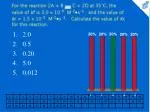For the reaction 2A + B {image} C + 2D at 35&#176;C, the value of kf is 3.0 x 10-3 M-2&#8226;s-1 and the value of kr = 1.5 x 10-2 M-2&#8226;s-1. Calculate the value of Kc for this reaction.

• 2.0
• 0.5
• 0.20
• 5.0
• 0.012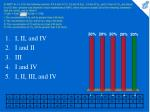At 990&#176;C Kc is 1.6 for the following reaction. If 4.0 mol of CO, 3.0 mol of H2O, 2.0 mol of H2, and 1.0 mol of CO2 are placed in a 5.0-liter container and allowed to reach equilibrium at 990&#176;C, which response includes all of the following statements that are correct, and no others? CO2(g) + H2(g) {image} H2O(g) + CO(g) I. The concentration of H2 will be greater than 0.40 mol/L. II. The concentration of H2O will be less than 0.60 mol/L. III. The concentration of CO will be less than 0.80 mol/L. IV. The concentration of CO2 will be greater than 0.20 mol/L.

• I, II, and IV
• I and II
• III
• I and IV
• I, II, III, and IV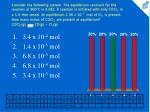Consider the following system. The equilibrium constant for the reaction at 900&#176;C is 0.082. A reaction is initiated with only COCl2 in a 1.0-liter vessel. At equilibrium 2.36 x 10-3 mol of Cl2 is present. How many moles of COCl2 are present at equilibrium? COCl2(g) {image} CO(g) + Cl2(g)

• 3.4 x 10-4 mol
• 1.4 x 10-5 mol
• 6.8 x 10-5 mol
• 6.8 x 10-4 mol
• 3.4 x 10-5 molThe equilibrium constant for the reaction N2(g) + O2(g) {image} 2NO(g) has the value 4.00 x 10-2. We introduce 28.0 g (1.00 mole) of N2 and 32.0 g (1.00 mole) of O2 into a 250-mL vessel and allow the reaction to reach quilibrium. What will be the equilibrium concentrations of all substances present? (In the answers, all concentrations are in moles/liter.) [N2] / [O2] / [NO]

• 0.91 / 0.91 / 0.18
• 3.02 / 3.02 / 0.98
• 1.82 / 1.82 / 0.36
• 0.36 / 0.36 / 0.72
• 3.6 / 3.6 / 0.72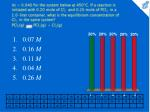Kc = 0.040 for the system below at 450&#176;C. If a reaction is initiated with 0.20 mole of Cl2 and 0.20 mole of PCl3 in a 1.0-liter container, what is the equilibrium concentration of Cl2 in the same system? PCl5(g) {image} PCl3(g) + Cl2(g)

• 0.07 M
• 0.16 M
• 0.11 M
• 0.04 M
• 0.26 M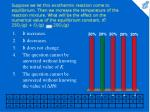Suppose we let this exothermic reaction come to equilibrium. Then we increase the temperature of the reaction mixture. What will be the effect on the numerical value of the equilibrium constant, K? 2SO2(g) + O2(g) {image} 2SO3(g)

• It increases.
• It decreases.
• It does not change.
• The question cannot be answered without knowing the initial value of K.
• The question cannot be answered without knowing the value of ΔH0.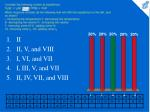Consider the following system at equilibrium. H2(g) + I2(g) {image} 2HI(g) + heat Which response includes all the following that will shift the equilibrium to the left, and no others? I. increasing the temperature II. decreasing the temperature III. decreasing the volume IV. increasing the volume V. removing some HI VI. adding some HI VII. removing some I2 VIII. adding some I2

• II
• II, V, and VIII
• I, VI, and VII
• I, III, V, and VII
• II, IV, VII, and VIII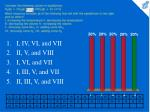Consider the following system in equilibrium. N2(g) + 3H2(g) {image} 2NH3(g) + 92.24 kJ Which response includes all of the following that will shift the equilibrium to the right, and no others? I. increasing the temperature II. decreasing the temperature III. decreasing the volume IV. increasing the volume V. removing some NH3 VI. adding some NH3 VII. removing some N2 VIII. adding some N2

• I, IV, VI, and VII
• II, V, and VIII
• I, VI, and VII
• I, III, V, and VII
• II, III, V, and VIII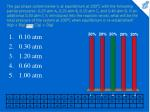The gas phase system below is at equilibrium at 200&#176;C with the following partial pressures: 0.20 atm A, 0.20 atm B, 0.10 atm C, and 0.40 atm D. If an additional 0.30 atm C is introduced into the reaction vessel, what will be the total pressure of the system at 200&#176;C when equilibrium is re-established?A(g) + B(g) {image} C(g) + D(g)

• 0.10 atm
• 0.30 atm
• 1.20 atm
• 0.60 atm
• 1.00 atm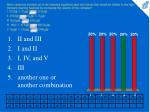Which response includes all of the following equilibria (and only those) that would be shifted to the right (forward reaction favored) by increasing the volume of the container? I. 2CO(g) + O2(g) {image} 2CO2(g) II. 2NO(g) {image} N2(g) + O2(g) III. N2O4(g) {image} 2NO2(g) IV. Ni(s) + 4CO(g) {image} Ni(CO)4(g) V. N2(g) + 3H2(g) {image} 2NH3(g)

• II and III
• I and II
• I, IV, and V
• III
• another one or another combinationConsider the following systems at equilibrium. Which response includes all the stresses (at constant volume) listed that would shift the equilibrium to the right (favor the forward reaction)? I. PCl5(g) {image} PCl3(g) + Cl2(g) &#8710;H = 92.5 kJ raise temperature II. 2SO2(g) + O2(g) {image} 2SO3(g) + 47.3 kcal lower temperature III. C(s) + H2O(g) + 131 kJ {image} CO(g) + H2(g) lower temperature

• II and III
• I
• I, II, and III
• I and III
• another one or another combinationConsider the following system at equilibrium. A(g) + 2B(l) {image} 2C(g) + D(l) + heat Which response includes all of the stresses listed that can result in new equilibrium concentrations of A that are less than the equilibrium concentrations before the stress was applied, and no others? I. increase pressure II. decrease volume III. decrease temperature IV. add more A V. remove some C

• I, II, and IV
• II, IV, and V
• I and III
• III and V
• none of theseFor the following reaction, &#8710;G0,298 is -277 kJ. Calculate the thermodynamic equilibrium constant, K, at 25&#176;C. R = 8.314 J/mol&#8226;K SiCl4(l) + 4H2O(l) {image} H4SiO4(s) + 4HCl(aq)

• 3.6 x 1048
• 1.6 x 1027
• 2.8 x 10-49
• 4.2 x 10-16
• 3.6 x 10-64